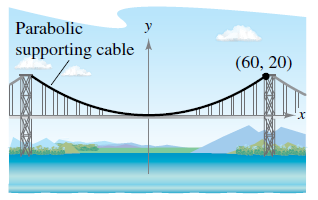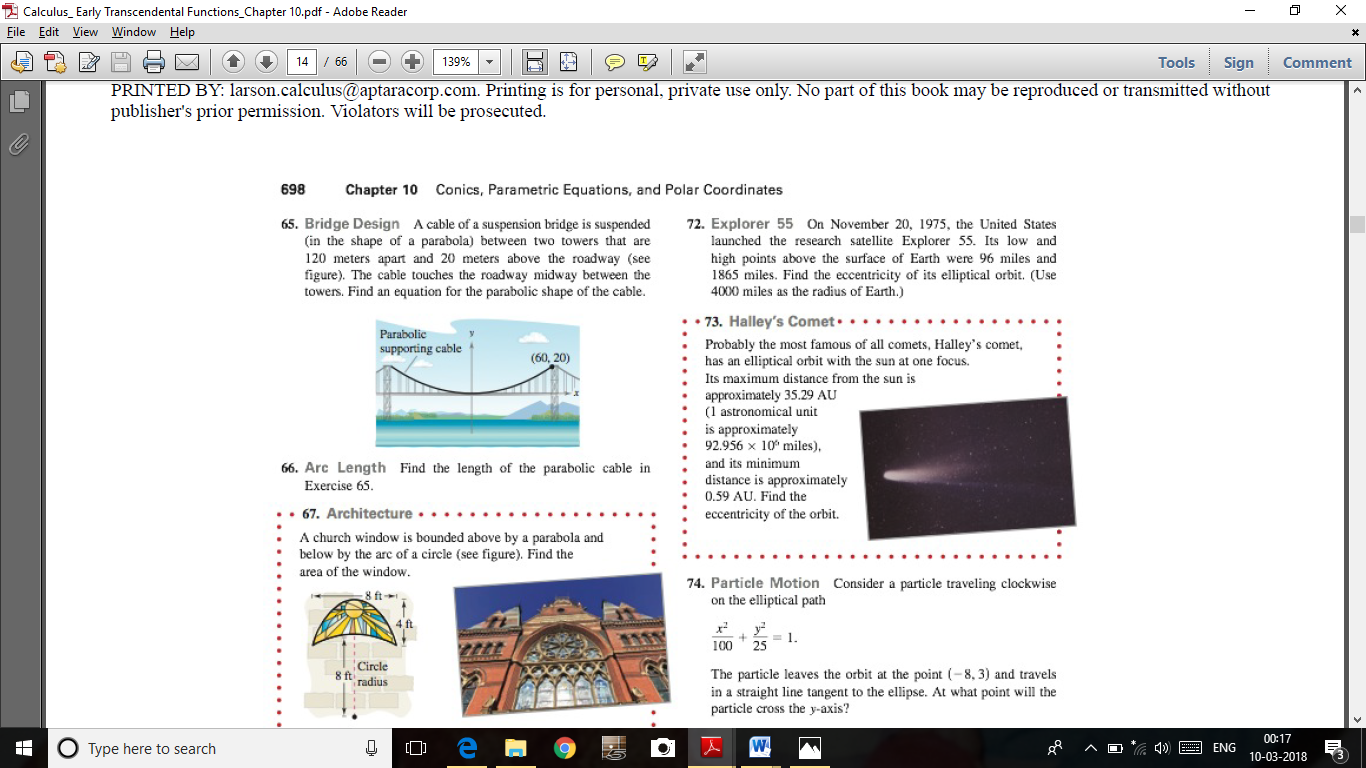Chapter 10.1, Problem 65E

Chapter
Section
Textbook Problem

Bridge Design A cable of a suspension bridge is suspended (in the shape of a parabola) between two towers that are 120 meters apart and 20 meters above the roadway (see figure). The cable touches the roadway midway between the towers. Find an equation for the parabolic shape of the cable.To determine
The equation for the parabolic shape of the cable for the below figure,Explanation

Given: Two towers which are 120m apart and 20m above from the roadway and the provided figure is,

Explanation:

The distance between the two towers is 120m.

That means, the point(60, 20) satisfies the parabolic equation x2=4py (because it

Still sussing out bartleby?

Check out a sample textbook solution.

See a sample solution

The Solution to Your Study Problems

Bartleby provides explanations to thousands of textbook problems written by our experts, many with advanced degrees!

Get Started

Evaluate the expression sin Exercises 116. (23)2

Finite Mathematics and Applied Calculus (MindTap Course List)

In Exercises 35-38, find an equation of the line that has slope m and y-intercept b. 36. m = 2; b = 1

Applied Calculus for the Managerial, Life, and Social Sciences: A Brief Approach

1340 Calculate y. y=sin2(cossinx)

Calculus (MindTap Course List)

Factor each expression in Problems 7-18 as a product of binomials. 14.

Mathematical Applications for the Management, Life, and Social Sciences

Divide: 15x65x4

Elementary Technical Mathematics